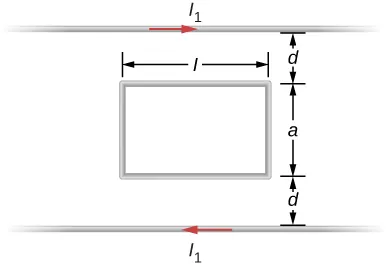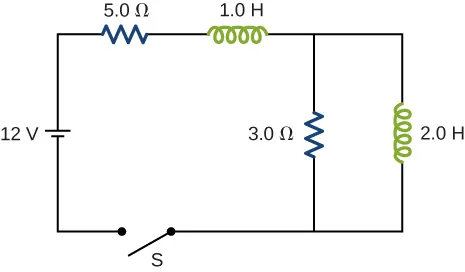University Physics Volume 2

73.

Show that the self-inductance per unit length of an infinite, straight, thin wire is infinite.

74.

Two long, parallel wires carry equal currents in opposite directions. The radius of each wire is a, and the distance between the centers of the wires is d. Show that if the magnetic flux within the wires themselves can be ignored, the self-inductance of a length l of such a pair of wires is

$L = μ 0 l π ln d − a a . L = μ 0 l π ln d − a a .$

(Hint: Calculate the magnetic flux through a rectangle of length l between the wires and then use $L=NΦ/IL=NΦ/I$.)

75.

A small, rectangular single loop of wire with dimensions l, and a is placed, as shown below, in the plane of a much larger, rectangular single loop of wire. The two short sides of the larger loop are so far from the smaller loop that their magnetic fields over the smaller fields over the smaller loop can be ignored. What is the mutual inductance of the two loops?76.

Suppose that a cylindrical solenoid is wrapped around a core of iron whose magnetic susceptibility is x. Using Equation 14.9, show that the self-inductance of the solenoid is given by

$L = ( 1 + x ) μ 0 N 2 A l , L = ( 1 + x ) μ 0 N 2 A l ,$

where l is its length, A its cross-sectional area, and N its total number of turns.

77.

A solenoid with $4x1074x107$ turns/m has an iron core placed in it whose magnetic susceptibility is $4.0×1034.0×103$. (a) If a current of 2.0 A flows through the solenoid, what is the magnetic field in the iron core? (b) What is the effective surface current formed by the aligned atomic current loops in the iron core? (c) What is the self-inductance of the filled solenoid?

78.

A rectangular toroid with inner radius $R1=7.0cm,R1=7.0cm,$ outer radius $R2=9.0cmR2=9.0cm$, height $h=3.0h=3.0$, and $N=3000N=3000$ turns is filled with an iron core of magnetic susceptibility $5.2×1035.2×103$. (a) What is the self-inductance of the toroid? (b) If the current through the toroid is 2.0 A, what is the magnetic field at the center of the core? (c) For this same 2.0-A current, what is the effective surface current formed by the aligned atomic current loops in the iron core?

79.

The switch S of the circuit shown below is closed at $t=0t=0$. Determine (a) the initial current through the battery and (b) the steady-state current through the battery.80.

In an oscillating RLC circuit, $R=7.0Ω,L=10mH,andC=3.0μFR=7.0Ω,L=10mH,andC=3.0μF$. Initially, the capacitor has a charge of $8.0μC8.0μC$ and the current is zero. Calculate the charge on the capacitor (a) five cycles later and (b) 50 cycles later.

81.

A 25.0-H inductor has 100 A of current turned off in 1.00 ms. (a) What voltage is induced to oppose this? (b) What is unreasonable about this result? (c) Which assumption or premise is responsible?

Order a print copy

As an Amazon Associate we earn from qualifying purchases.